# In the circuit of Figure P6.9:R = 1.3 k2R2 = 1.9 k2C = 0.5182 µFDetermine:a. How the voltage transfer functionV.(jo)V.(jo)Hy(j») =behaves at extremes of high and low frequencies.b. An expression for the voltage transfer function andshow that it can be manipulated into the formH.H,(jo) =TFjf(@) whereR2H. =f(ω) -wR¡R2CR + R2R1 + R2c. The frequency at which f(@) = 1 and the value ofH, in decibels.1,(j) R,I(ja)wwV(jo)c+R V(ja)

Question
2 views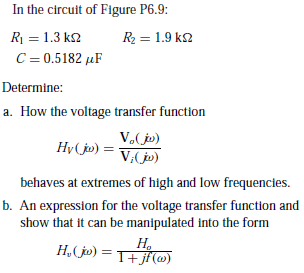help_outlineImage TranscriptioncloseIn the circuit of Figure P6.9: R = 1.3 k2 R2 = 1.9 k2 C = 0.5182 µF Determine: a. How the voltage transfer function V.(jo) V.(jo) Hy(j») = behaves at extremes of high and low frequencies. b. An expression for the voltage transfer function and show that it can be manipulated into the form H. H,(jo) =TFjf(@) fullscreen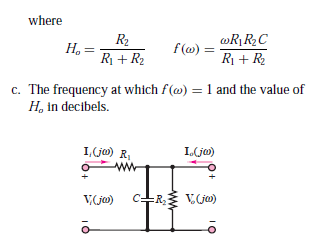help_outlineImage Transcriptionclosewhere R2 H. = f(ω) - wR¡R2C R + R2 R1 + R2 c. The frequency at which f(@) = 1 and the value of H, in decibels. 1,(j) R, I(ja) ww V(jo) c+R V(ja) fullscreen
check_circle

Examination of the given circuit.

The circuit is shown below.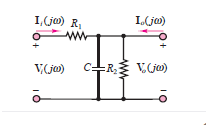First, the transfer function will be determined in order to solve the first subpart. Once the value of the transfer function is calculated the other subparts can be subsequently determined.

(a) Calculation of the transfer function.

In the given circuit, the capacitor and the resistor are connected in parallel. First be calculate the equivalent impedance after that the voltage divider rule will be applied to calculate the output voltage, thus the value of the transfer function can be determined.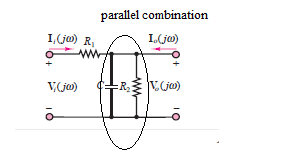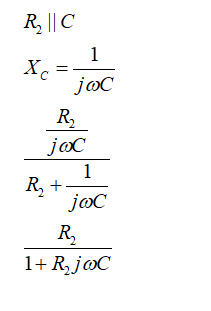The output voltage is given as: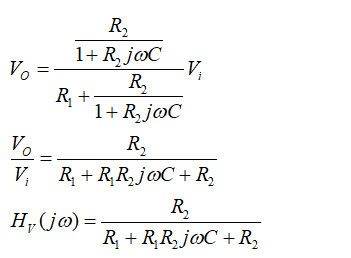The transfer function of the given circuit is calculated, the behavior of the transfer function at low frequency is given as: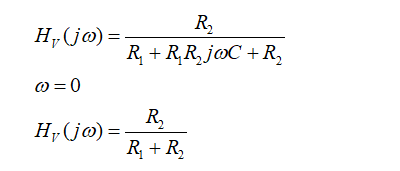At low frequency, the transfer function comes out to be: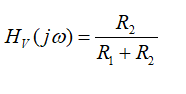The behavior of the transfer function at low frequency is given as: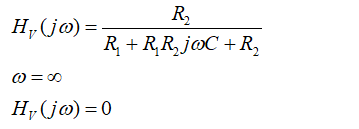At very high frequency the transfer function comes out to be zero.

(b) Part

To proof that the transfer function can be represented in the form as: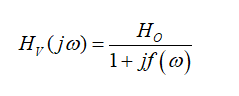The expression of the transfer function will be divided by the suitable parameters of the circuit to achieve the desired expression.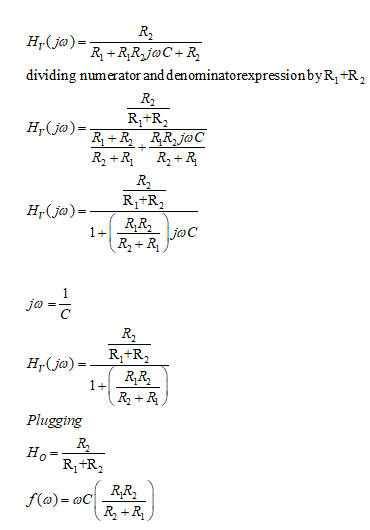The above transfer function becomes.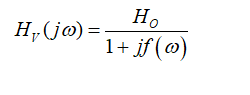...

### Want to see the full answer?

See Solution

#### Want to see this answer and more?

Solutions are written by subject experts who are available 24/7. Questions are typically answered within 1 hour.*

See Solution
*Response times may vary by subject and question.
Tagged in